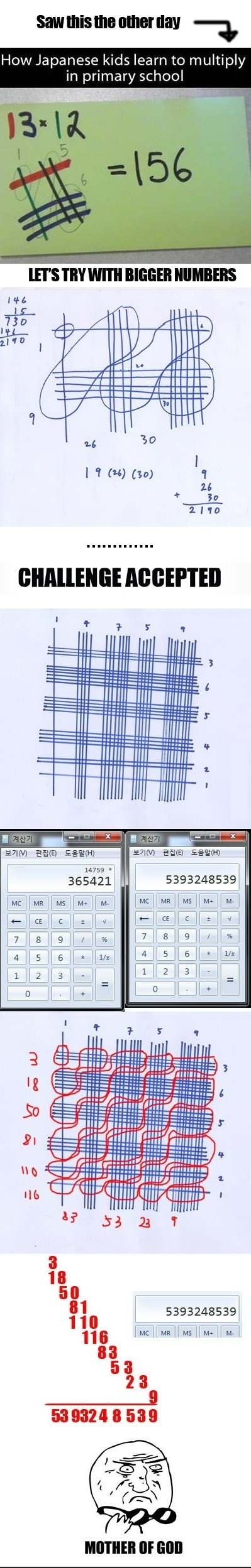WeÃ¢â‚¬â„¢ve all had our lessons in Mathematics in schools and colleges and what haunts us bad still is multiplication of long serpentine trail of digits. But how would your reaction really be if you get to know that what still comes to haunt you back in Maths is a cakewalk for kids (as young as 8) in Japan?

Obviously, you canÃ¢â‚¬â„¢t stop cursing yourself. But save that for a while to know what these little Japanese versions of AryabhattaÃ¢â‚¬â„¢s do to make multiplication problems look like a thing of kids.

So, what will you do to simply multiply 13 with 12? Undoubtedly, you will follow the pattern that was taught to you in schoolsÃ¢â‚¬â€multiply 2 with 3 and 1 and then multiply 1 with 3 and 2. After that you will sum up the results.

Exactly, thatÃ¢â‚¬â„¢s the catch here. Japanese kids donÃ¢â‚¬â„¢t follow this traditional Indian method of multiplication in Math. They are taught to do this in very different way by their teachers. They find this method really helpful and swift for multiplications of really huge numbers.

So, what they really do is draw lines. Yes, draw lines to multiply! LetÃ¢â‚¬â„¢s take the same example once again.

We got 13 to multiply with 12.

There are two numbers in this problemÃ¢â‚¬â€13 and 12.

LetÃ¢â‚¬â„¢s take the first numberÃ¢â‚¬â€13.

Draw a vertical line for its first digit 1 and 3 vertical lines for another digit 3 with enough space in-between.

Remember that the number to be multiplied (in this case 13) is depicted by vertical lines always.

Now letÃ¢â‚¬â„¢s take another numberÃ¢â‚¬â€12.

Draw 1 horizontal line on the top for its first digit 1interesecting all four vertical lines. Then, draw 2 more horizontal lines, maintaining enough space from the first horizontal line, for the numberÃ¢â‚¬â„¢s another digit 2 again intersecting all four vertical lines.Now, count all the diagonally opposite intersections starting from the point where least number of intersections has been made.
Refer to the images below for more clarity.

You will get 1, 5, and 6 intersecting points. Now, combine them to get the answer. Of course, your answer will be 156.To multiply numbers with 3 digits, you apply the same rule of drawing vertical and horizontal lines intersecting each other and counting total numbers of diagonally opposite intersections.

But here you add these numbers of intersections as shown in the second image above. Whew! If you are inspired by this weird but fast method of multiplying numbers then try it on your own peril, because this Japanese method can prove really complicated for multiplying huge numbers.